Select Page

### Beats to the Bible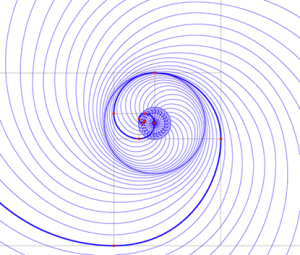This is the final part of our 4 part series on the Cosmic Frequency and it is centered on our DNA and the 20 amino acids that comprise our genetic make-up. There is a beat to the heart of the Fibonacci Numbers, and it translates to the Torah.  This is like the Bell Numbers that are fully integrate into the Torah through the extremely rare Bell Prime Indices (2, 3, 7, 13, 42, 55 and 2841), such as in the Path of the Vav (ו) found in the Shema (134255). As you may recall there are 13 Vav (ו) initials in the first 42-word paragraph of the Shema, and 42 in the rest of the Shema, making 55 in total.   Moreover, the first 6 of the Bell Prime Indices (2 + 3 + 7 + 13 + 42 + 55) add up to 122, as in the 122 words in the middle paragraph of the Shema that came straight out of Devarim 11:1321.

Of course, the first 6 of the 7 Bell Prime Indices is perfectly aligned with the Fibonacci Numbers, in that 2 is 2; 3 is 3; (1 + 1 + 2 + 3) = 7; (1 + 1 + 2 + 3 + 5) = (2 + 3 + 7); 13 is 13; (8 + 13 + 21) = 42; and (21 + 34) = 55.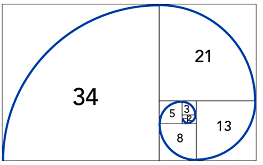In combinatorial mathematics, the Bell Numbers count the possible partitions of a set.  To understand partitioning, we can see that One element can be divided only 1 way, making 1 the first Bell number. Two elements can be split up as (1,2) and as (12) or two ways, making 2 the second Bell number. Three elements can be split or partitioned into (1,2,3); (12,3); (1,23); (13,2); and (123) or 5 ways, making 5 the 3rd Bell number. In other words, the nth of these numbers, Bn, counts the number of different ways to partition a set that has exactly n elements.

The 6th Bell Number is 203, the value of the first Triplet (ברא) in the Torah.

If you rank all the Bell Numbers from B1 to Bn and record where those 7 rare Bell Primes are found, you have an index of all the Bell Primes. This is known as the Bell Prime Indices.  The Indices of the 7 known Bell Primes are the 2nd, 3rd, 7th, 13th, 42nd, 55th, and 2841st Bell Numbers, giving us the so far finite sequence: 2, 3, 7, 13, 42, 55 and 2841. This sequence is called “the Indices of Prime Bell Numbers.” Our supercomputers have only found 7 Bell Primes so far, making them exceedingly rare in the universe.

The 7th of the Bell Numbers and 3rd Bell Prime is number 877, and the 7th Bell Prime Index is 2841. In the 7 Days of Creation there are 10 Utterance by the Creator, 9 of which begins with “Vayomer Elohim,” connected to the 42-Letter Name, followed by a command to the elements of hyperspace. The sum of those 9 commands is (232 + 405 + 122 + 705 + 666 + 606 + 497 + 425 + 60) = 3718, which equals (877 + 2841).  Add to that the 7 tiers of the 42-Letter Name, and we have (3718 + 42) = 3760, the Spiritual Time component of Spherical Time, corresponding to the 7 dimensions of Zeir Anpin.

Partitioning is a mathematical concept, not a specific number or constant, but it does generate sets of specific numbers. It is a form of counting and in that sense, it is a cipher that can be applied to items, any items from atoms to stars. As we saw the importance of the narrow subset of Bell Primes, it must be noted that the 10th Partition Function Number is 42, and the 28th is 3718.

The Bell Numbers have to do with combinatorics and integer sets, and so are the Fibonacci beats, but is far simpler than it sounds because the combinations are of only 2 beats, a short one and a longer one that is twice the short one.

Right away that 2:1 proportion reminds us of the golden proportion based on Phi (φ), so it is not surprising that it is related to the Fibonacci Numbers.  For any given integer there is a specific maximum number of combinations of these beats that will equal it. In a set of 1, only 1 short beat will fill the bill. On a set of 2, we could have either 2 short beats or 1 long one, thus there are a total of 2 possible combinations. With 3, we get 3 possibilities (3 short; 1 short, 1 long; or 1 long followed by 1 short). With 4, there are now 5 possibilities: we could add a short beat to each of the prior set to get (4 short; 1 short, 1 long, 1 additional short one; or 1 a long followed by 2 short) and there are 2 other new possibilities (2 long; or 2 short and 1 long). The pattern holds for every subsequent number, building on the previous two, so that they follow the Fibonacci sequence: 1 for 1; 2 for 2; 3 for 3; 5 for a bounded set of 4; 8 for one of 5; 13 for 6; 21 for 7; 34 for 8…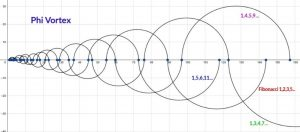When we apply these beats to the Torah as a cipher, we see that the first verse of Genesis 1:1 has a Fibonacci value of 42 beats.

When applied to the first 8 words of the Torah that reflect Binah and the Essential set of the 11 Triplets of Creation, they have a value of 50, like the 50 Gates of Binah.  It should also be noted that the 33 letters in the 11 Triplets of Creation reflect the first 7 Fibonacci Numbers (1 + 1 + 2 + 3 + 5 + 8 + 13) = 33, and that they total 3003, the 77th Triangular Number.

The Torah’s first 42 Letters or 11 words, which are derived from the 42 letters in the 42-Letter Name, have a value of 60, as in the hidden letter Samech (ס) concealed in the center of the Name.

Meanwhile, the 14 words of the Torah’s 2nd verse have a Fibonacci value of 67 beats as in the value of the sefira and dimension of Binah (67), while the first 5 verses that comprise Day One have a collective Fibonacci beat value of (42 + 67 + 30 + 59 + 62) = 260, as in the YHVH (יהוה), and as in the constant 260 of the 8 x 8 normal magic square.

When the beats are added to the Torah’s 7-word first verse, its numerical value becomes (2701 + 42 + 7) = 2750 or 100 x 27.5 Hz, the Primal Frequency. Once again, the 5 Books of Moses demonstrating the interaction of the field of Phi (137.5o) with the Primal Frequency (27.5o), as in 137.5/27.5 = 5.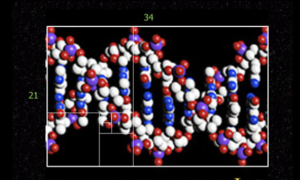These Fibonacci beats are also the beats of life, as our DNA also conforms to it in multiple ways.  One frequency, one harmonic pattern; everything either conforms to it, or it is crumbled up and destroyed, recycled.

### Our DNA and the Frequency of the Cosmic Consciousness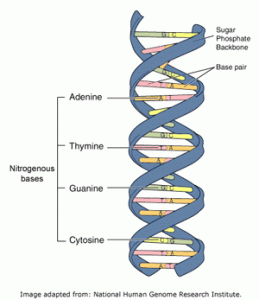The 4th root of the Torah’s total number of words, letters, and verses is exactly 25, and the average of the first 25 Prime Numbers is exactly 42.4, as in Moshiach Ben David, the Cosmic Consciousness. While the collective value of the collective values of those 25 Prime Numbers, in other words, the 25th Tetra Prime, is exactly 8600, as in Elohim (אלהים), the 42.4-value aligns them with the sum of all the 22 Names of the 22 Hebrew letters, 4248 and with the 22 square roots of those Names that total 424.242, which in turn aligns them with the 42 letters of the 11 dimensions of the Tree-of-Life, whose 3 initials of its central core (Keter-Da’at-Tiferet) total 424, just as do the 4 nucleotides (ACGT) of our DNA in Hebrew (אכגת).

No one purposely came up with the names of the 4 nucleotides of DNA so that their initials would equate to 424. All 5 nucleotides in DNA and RNA are named for their nitrogenous base and those bases were each named independently and individually for random reasons. For example, in 1885 a brilliant scientist, Albrecht Kossel, named adenine after the Greek word for pancreas, ( ἀδήν “aden”) from which his sample had been extracted, and Guanine was named after guano, bird droppings, from which the chemical was first isolated.  These are very random origins, or alternatively, Divine inspiration within intelligent design, just like all the units of measure, the temperature scales, the physical and metaphysical dimensions of our cosmos, and the dates in history.

There are 3.3 billion pairs of nucleotides that form the 46 chromosomes of our DNA (nucleic acids), and all are comprised of the 4 nucleotide bases that code for the 20 amino acid chains of our proteins. Those 4 bases are adenine (א), guanine (ג), cytosine (כ) and thymine (ת), collectively (אגכת) or 424, Moshiach Ben David, the Cosmic Consciousness.

With their ordinal values they have a complete numerical value of 460 as in 10 x 46 Chromosomes and as the 54th Triplet (נית) of the 72 Triplets, whose ordinal value is also 46.  The value 54 that keeps cropping up, like the 54 Portions of the Torah, is also the sum of the first 8 Fibonacci Number (1 + 1 + 2 + 3 + 5 + 8 + 13 + 21) = 54, that match each set of major and minor grooves in our DNA and Chromosomes.

And like the 3-letter root or Triplets in the Hebrew language, the language of DNA is arranged in 3-letter codes made up of these 4 letters (אגכת). Three (3) represents the letter Gimmel (ג) of, and the 3 letters ACT (אכת) are the only 3 letters that conformed perfectly to the alef-bet polynomial function curve.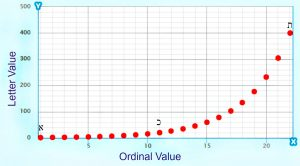The 4 DNA base pairs are matched 2 x 2 with adenine being paired only with thymine, and cytosine only with guanine, in order that they each have 3 organic rings in their composition which causes them to spiral along a balanced axis. The complete gematria value of their representative letter pairs is (1 + 400 + 1 + 22) = 424 for the (adenine – thymine) pair, and (20 + 3 + 11 + 3) = 37 for the (cytosine – guanine) pair, as in the inner or ordinal value of Chochma, the 2nd highest sefira, that of Wisdom.

Like the recurrence value pairs—the number of times a specific word value is found in the Torah—of (27 with 83) and (28 with 82) that each added up to 110, the inner and outer values for Chochma, (37 with 73), also add up to 110. Of course, the Torah’s first verse’s value of 2701 is their product (37 x 73).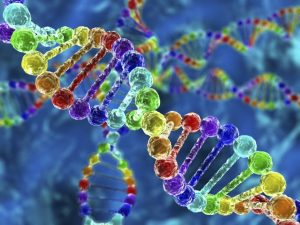This is also like our 46 Chromosomes and 64 Codons in our DNA that also equal (46 + 64) = 110, which all align with (4 x 27.5), the Primal Frequency and first major interval. Then again, maybe this is all just a miracle since 110 is the value for the Hebrew word “nes (נס) miracle,” implying that being aligned with the Primal Frequency of the aether is magical and akin to miracles.

The 64 Codons in our DNA align with the complete value of the Ein Sof (אין־סוף), 1660 that is equivalent to (64 x 27.5). It is the Primal Frequency Sourced from the metaphysical Endless, the Infinite and the Pure Essence of G-d. It makes sense since 64 is the value of Adam and Eve, (45 + 19) = 64, and Adam (אדם) is an acronym for Adam-David-Moshiach, the path that traces the Spherical Time Clock.

The value 1660 is also the value of the prophetic end of days verse from Malachi 3:23, which aligns with 64 being the value of din (דין), judgement, a permutation of the 50th Triplet (דני) of the 72 Triplets, as in the 50th and final gate of negativity where the world is at currently and where we were at the time of the Flood and just prior to the Exodus from Egypt.

### הִנֵּה אָנֹכִי שֹׁלֵחַ לָכֶם, אֵת אֵלִיָּה הַנָּבִיא–לִפְנֵי, בּוֹא יוֹם יְהוָה, הַגָּדוֹל, וְהַנּוֹרָא.

• #### – Malachi 3:23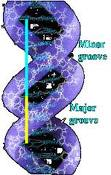Our DNA twists into major and minor grooves. These are defined by the Phi (φ) proportion Then within each set of these grooves, in other words within one full turn of the DNA helix, there are 10.5 nitrogenous base pairs. This too is in the Phi (φ) proportion with the value 27.5, as (10.5 + 17) = 27.5, and as 17/27.5 = 1/φ. Meanwhile, the 34o rotation per base pair and the 34 Å (angstrom) long stretch for that full turn is (2 x 17), as in the 9th Fibonacci Number, along with the constant (34) of the 4 x 4 normal magic square.While it makes perfect sense for our DNA to follow the Phi (φ) proportion the way so many other aspects of life do, it is beyond reason that the average weight of those 4 nucleotides is equal to 499.5 g/mol, as in the value of the 27 letters of the Alef-bet, 4995.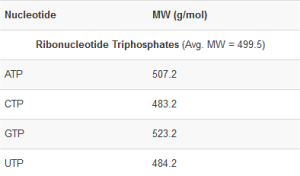Even more extraordinary is that the total for the 4 nucleobases, Adenine (135.13), Guanine (151.13), Thymine (126.11), and Cytosine (111.1) divided by their initials (424) is 1.23456789, not to mention the obvious individual nucleobase connections to the Networks of One (1), as in Echad (13) for Adenine and Alef (111) for Cytosine; and to Ehyeh (אלף־הה־יוד־הה) of value 151 for Guanine; and to the Essential Cube whose faces and planes each total 126 for Thymine.  Man thinks he named these building blocks and created the weight system, but the numbers were there long before Man.  Man only followed the Divine forces and paths of the cosmos that led him to them.

The sum of the 27 letters in the Alef-Bet, 4995, or alternatively the average of the 4 nucleotides (499.5) divided by the Prime Frequency (27.5 Hz) is 181.63636, as in the 42nd Prime Number (181) and as in the sum the first 42 digits in the set of Prime Numbers that is also 181. While the sum of the digits through 181 (the 42nd Prime Number) is 378, the 27th Triangular Number, the value 378, or (9 x 42), is the total ordinal values of the 27 letters whose standard value is 4995. The value 378 is also (18 x 21), the 8th Fibonacci Number, which numerically translates using the cosmic interface to Chai, life, times the higher Name Ehyeh (אהיה).

These cosmically align with the Network of One through the 181 digits in 111! (factorial) with 111 being the numerical value of Alef (אלפ), One (1) and of the nucleobase Cytosine (111.1). We must keep in mind that while it only mattered to the Cosmos and the Creator what names were given to the nucleotides, if any of these atomic weights were altered in the slightest Man may never have existed.

This connection to One (1) and the Primordial Alef (אלפ) also has to do with the relationship between Phi (φ), the concept of 5, and the Primal Frequency (27.5 Hz). Whether we are talking about the 2nd Triplet (יתץ) or the 14th Triplet (צית) in the 42-Letter Name Matrix that each equal 500, or the 10 remainders that total 5 or 5000, their Quotient (27.5) is 500/27.5 = 18.18 as in 181, and as in the 3 iterations of the Name Alef (אלפ) that equal 1818, and as in Chai, life, whose square root is 4.2426, the Cosmic Consciousness, like the 4 nucleotides in our DNA.

### 20 Amino Acids

Like the dynamic between the Numbers and the Hebrew letters, our DNA and RNA are the blueprints and messaging interface. In the case of physical life, these interface with exactly 20 amino acids to produce the necessary proteins that create our bodies and systems. While these align metaphysically with the Tower of Truth’s 20 stages of stacked cubes, they also align with the Torah’s first verse and with the Names of Binah to which that Tower reaches.

The molecule weight of the 20 Amino Acids in our DNA is 2738.01 g/mol, and the value of the Torah’s first verse (2701) along with its reciprocal (1/2701), also known as the 42-Letter Name, is (2701 + 37.01) = 2738.017, which is 2 x (372).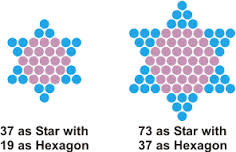The inner or ordinal value of the 4 nucleotides Adenine (א), Guanine (ג), Cytosine (כ) and Thymine (ת), collectively (אגכת), is 37, the same as the ordinal value of the sefira of Chochma (Wisdom). While the inner and outer values of Chochma (חכמה) are 37 and 73, whose product is (37 x 73) = 2701, the value 37 is the difference that evens out the 7 words of the Torah’s first verse with the sum of the 20 amino acids, 2738.  And as Glen recently pointed out in a comment, (3 x 7 x 7 x 3) is 212 or 441, as in Emet (אמת), Truth, and the 20 stage Tower of Truth.

It is not a coincidence then that Joseph was sold by his brothers for 20 pieces of silver in chapter 37 within paragraph 73 of Genesis, in one of the most pivotal moments of the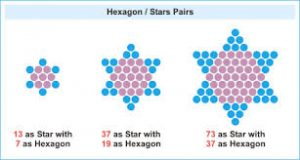Torah and of history to begin the 210 years of exile, the 20th Triangular Number in the schematic of the Tower of Truth. It is also not a coincidence that the 210 cu height of the Tower of Truth is 481 feet or (13 x 37).

Moreover, while 2701 is the 73rd Triangular Number and consists of 28 letters, the atomic total number, 2738, has 73 at its core (2738) and 28 at its periphery (2738), or that its 4 digits sum to (2 + 7 + 3 + 8) = 20, or that their product equals 336, as in the 336 letters in the 112 Triplets that align with the 112 chakras in the human body.

Meanwhile, the complex gematria cipher that utilizes the first 27 digits in Pi (π), the Prime Numbers, and the Ordinal values when applied to the 3 expanded iterations of the Higher Name Ehyeh (אהיה) equals 2738, once again matching the molecule weight of the 20 Amino Acids in our DNA, 2738.01 g/mol.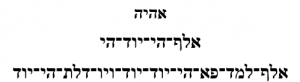Coincidence or not, Tyrosine, has a molecular weight of 181.18… with its connections to 42 and the Prime Numbers just enumerated.

## Amino acids: formula and molecular weight

 Amino acid Chemical formula Molecular weight, g/mol Isoleucine C6H13NO2 131.1736 Leucine C6H13NO2 131.1736 Lysine C6H14N2O2 146.1882 Methionine C5H11NO2S 149.2124 Phenylalanine C9H11NO2 165.1900 Threonine C4H9NO3 119.1197 Tryptophan C11H12N2O2 204.2262 Valine C5H11NO2 117.1469 Arginine C6H14N4O2 174.2017 Histidine C6H9N3O2 155.1552 Alanine C3H7NO2 89.0935 Asparagine C4H8N2O3 132.1184 Aspartate C4H7NO4 133.1032 Cysteine C3H7NO2S 121.1590 Glutamate C5H9NO4 147.1299 Glutamine C5H10N2O3 146.1451 Glycine C2H5NO2 75.0669 Proline C5H9NO2 115.1310 Serine C3H7NO3 105.0930 Tyrosine C9H11NO3 181.1894

The 20 amino acids that comprise our DNA can be arranged in a 4 x 5 matrix according to their properties, whereby the first column’s molecular weights (Asp, Arg, Gln, Hus, Glu) add up to 755.7782, a measure that alludes to the Spherical Time event horizon, 5778 and to the base of the Pyramid of the Sun in feet.

Nevertheless, the 4 columns astonishingly conform perfectly to the Torah’s first verse. The first 5 words of that verse equal (3 x 666) and the final 2 words equal 703 which compares to the 20 amino acid matrix in which the 1st and 4th columns equal (2 x 703) and the 2 middle columns equal (2 x 666). It breaks down to (2 x 18 x 37) + (2 x 19 x 37) vs the Torah’s (3 x 18 x 37) + (19 x 37) or (74 x 37) vs (73 x 37).

This gets more impressive as we go on, yet already the only way for this symmetry to occur between the essence of life and the Torah is for them to have the same Creator.

### (73 x 37 = 2701)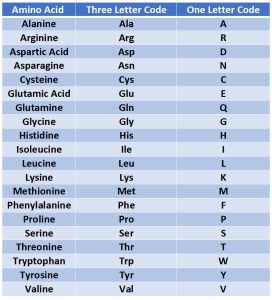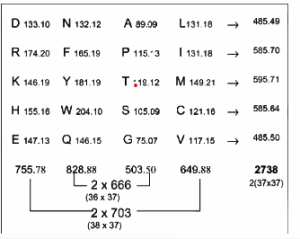The matrix is arranged in a way such that the molecular masses of the 5 row totals without their decimal places from top to bottom lay out symmetrically as 485, 585, 595, 585, 485, while the differences between them are likewise symmetrical: 100, 10, 10, 100, and add up to 220 or (8 x 27.5). The middle column in this chart by Miloje M. Rakočević represents the number (n) of nucleons in the sidechains of the amino acids in the rows. We see the same pattern of symmetry as in their molecular masses and the same differential sum of (8 x 27.5).

By the way, the symmetry holds whether the molecular mass is truncated as in Rakočević’s original charts or rounded as in the current one. The corresponding English symbols for the remaining three columns are (Asn, Phe, Tyr, Trp, Lys, Ala, Pro, Thr, Ser, Gly, Leu, Ile, Met, Cys, Val). And the first 2 columns vs the last 2 columns split the 2738 total into the same 5842% proportion as the even/odd letters in Alef-bet are split into, and Adam and Eve proportion as well (19/45 and 26/45).

The rest of the molecules’ nucleons are found in their amino acid functional groups and each row has exactly 296 of them, as in the value of the 7th word inthe Torah’s first verse, H’erz (הארץ), meaning Earth and “the land”, as in Adam was made from the dust.  The full phrase in that verse of (73 x 37) is V’Et H’Ertz (ואת־הארץ) of numerical value 703 or (19 x 37), 26.0% of 2701, also like Adam and Eve.

The interesting thing besides the symmetry in the number of nucleons is that their square roots belie the imprint of the cosmos on the 20 amino acids. The first and fifth rows total 189 and the square root of 189 is 13.75, which is both the Primal Frequency 27.5/2 and the Phi (φ) angle (137.5o). The square root of 289, as in the second and fourth rows, is 17, which 27.5/φ, and the square root of the middle row, 299, is 17.29, as in the 1729 recurrences of the value 26 in the Torah.

Meanwhile the sum of those 5 square roots is 78.78.., as in the 7878 initials and final letters Bet (ב) in the Torah, and as in (6 x 1313), which reflects the year 1313 BCE (2448 HC) when we received the Torah, and the polynomial constant 1.313 that the Alef-bet is based on (x1.313). It also reflects the volume of the sphere whose circumference is 360” or 13.0 cubits.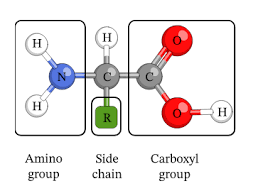Now, while the total of the 3 different sums (189, 289, 299) of the sidechain nucleons is 777, or (21 x 37) the differences between them and the 296 number of their functional groups or heads, is (107, 7, and -3), which adds up to 111, or (3 x 37), aligning them with all the connections to the Alef (אלף) or One and with the design of our Solar system. The net total difference for all 5 nucleons is 225 or 152.

The total value of the 3 different sums (485, 585, 595) of the molecular masses is 1665, which is also (45 x 37 or 15 x 111) that seems to mimic the 777 = (7 x 111) sum of the nucleons and their net 152-difference between them.  There is also a correlation with the constant 15 of the 3 x 3 normal magic square and the 111 constant of the 6 x 6 normal magic square. Moreover, this also means that the 1665 molecular mass less the 777 sidechain nucleons equal (1665777) = 888, as in (8 x 111) for the 3 corresponding nucleons found in their amino acid functional groups.

This is also the same 1665 that we saw in the 3 rows (618, 753, and 294) of that 3 x 3 normal magic square and in the each of the 3 tiers of the 33 normal Essential Magic Cube of Creation (42), and thus in 1/3 of the 4995 total of the Alef-bet and of the average weight of the 4 nucleotides (ATCG), 499.5 g/mol.

The other 2 of the 5 rows of molecular masses of the amino acid matrix equal (485 + 585) = 1070, or 107 x 10, like the 2 differences between 296 and 189.

The 2 row sums of 585 may also align with the 62.5%/37.5% split in the 2 spheres of the Tree-of-Life. Since 62.5% equals 225o when added to the 360o of the lower sphere, we get a total of (225o + 360o) = 585o.

Moreover, the 2 rows that equal 585 total 1170, as in the 11 sefirot (dimensions) and 70 branches of the Tree-of-Life. Furthermore, since the 4 x 5 amino acid matrix totals 2735, when we subtract the 2 rows that equal 585 from it, we get (27351170) = 1565, as in the katan string value of the YHVH (יהוה).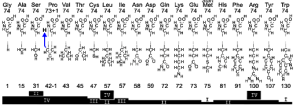In the chart above we see that the core block or functional group of each of the 20 amino acids consists of 74 nucleons or (2 x 37), which is (40 x 37) in total. The one exception is Proline that includes a 1 nucleon transfer, which is significantly enough from its 42-nucleon sidechain to its 73-nucleon core. The numbers in the 20 sidechains as they stretch from 1130 are significant as well, including 15, 31, 45, 72, 73, 91, and 107 to name the most prominent.  So how extraordinary is it that the log (20) = 1.30103!

The 20 sidechains total 1255, giving us a difference between them and the functional groups of (14801255) = 225 or 152 again, like the Name Yah (יה) and like the constant 15 of the 3 x 3 normal magic square.  The 74 core nucleons in each of the 20 amino acids align directly with their (74 x 37) total molecular weight, which also means that the sidechain molecular weight is (7440) = 34 x 37, as in the constant 34 of the 4 x 4 normal magic square.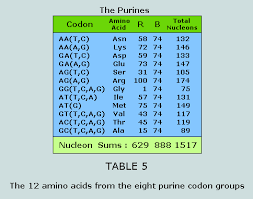There are various non-arbitrary ways to split the amino acids into specific groups, including opposing or pairing the codons along their Rumer’s transformation and half-transformation (T – C, A – C) vs (T – A, A – G). When we do this (as illustrated) below, the side chains balance is 703 or (19 x 37) in each half and 1665 = (666 + 999) = (18 x 37) + (27 x 37) nucleons for the whole molecules for each side as we saw above. There are multiple other ways to logically parse the amino acid and codon groupings as multiples of 37, including separating Purine and Pyrimidine as like bases (as illustrated).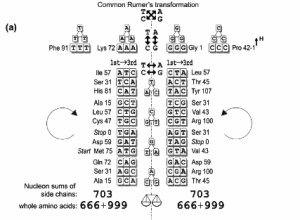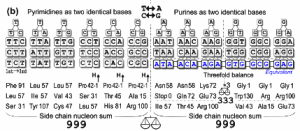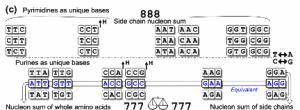And still others…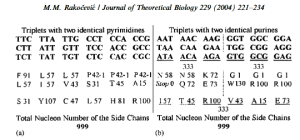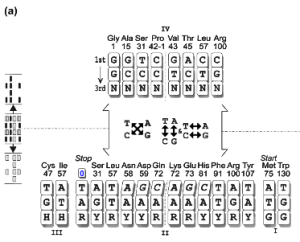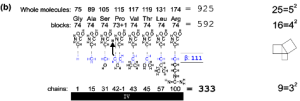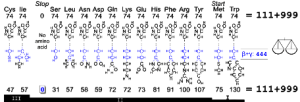Bottom line, as the authors of this analysis first proposed, they are organized by intelligent design and not random or even guided evolution as the in the “alien hypothesis.” And as we have seen, our DNA blueprints and amino acid building blocks were designed and constructed by the same Creator as the Torah, the Alef-bet, the Cosmos, and all the mathematical fields and networks that comprise them.

As Vladimir shCherbak has proven, it is beyond all statistical probability that the value and concept of 37 has been intelligently designed into our DNA and our amino acids and into the Torah, including into the ordinal position of the 37 letters in the first 9 words of the Torah that total 703 or (19 x 37), just like the  (19 x 37) value of the Torah’s 6th and 7th words that represent the Earth and physicality.

The value 45 is that of Adam (אדם), Man, from the amino acid equation (45 x 37) = 1665 in the 3 rows of the amino acids; and of the 3 x 3 normal magic square; and from the equation (45 x 111) = 4995, as in the total of the Alef-bet, which is like the average weight of the 4 nucleotides (ATCG), 499.5 g/mol. And the value 19 is that of Eve, Chava (חוה).The difference between Adam and Eve is (4519) = 26, as in the YHVH (יהוה). Together Adam (אדם) and Eve (חוה), the physical source of our DNA, equal (45 + 19) = 64, as in the 64 codons of our DNA, and 64/37 = 1.73, as in the gematria katan value of the 42-Letter Name, the Source. The inverse or ratio 37/64 = .5781, as in the year 5781 HC or 2021 when Man played G-d with our DNA.

Of course, (37 + 64) = 101, the value of the 53rd Triplet (ננא) in the 72 Triplet Matrix and the 26th Prime Number, as in the YHVH (יהוה). The value 101 is also the sum of the 3 duplicate Names (והו), (לאו), and (מבה) among the 72 Triplets, who’s values also happen to be Prime Numbers, as in (17 + 37 + 47) = 101. Combining two of them they reform into the equation (37 + 64) = 101.  These 3 duplicates are first presented as the 1st, 11th, and 14th Triplets, as in (1 + 11 + 14) = 26. And since (101 + 26) = 127, the 31st Prime Number, which brings us back to the Perfect Numbers and the 7 words of the Torah’s first verse that can recombine in exactly 127 possible ways with each of those words utilized exactly 64 times, in the same way that the numerical word value 127 is utilized exactly 64 times in the Torah, aligning them with the associated 127 and 64 ranks of the Triangular (8128) and Hexagonal Numbers (8128) that compose (127 x 64) = 8128, the 4th Perfect Number, and the 64 Codons of life. By the way, the initials of those 3 Triplets have a complete value of (76 + 31) = 107, as in the 107th Triangular Number, 5778, the Event Horizon, and their 3 final letters form the first of the 72 Triplets (והו).

The preponderance of the connections to 37 in our DNA, like the 1147 or (31 x 37) atoms in the 20 amino acids, and the Torah is also reflected in the 37 letters Vav (ו) in the concealed 112 Triplets of the Torah. The letter Vav (ו) represents the 6 bundled dimensions of Zeir Anpin, the link between physicality and the spiritual, which may be why those 37 letters Vav (ו) equal 222, as in (6000222) = 5778. And as in the complementary second set of duplicates—the 49th, 17th, and 55th Triplets—whose ordinal placement and value is (49 + 17 + 55 + 101) = 222.

Oddly enough, those 1147 or (31 x 37) atoms align with the 12 sons of Jacob in 3 different ways.

#### Benjamin (בִּנְיָמִן), 162; Dan (דָּן), 54; Yisaschar (יִשָּׂשכָר), 830; and Zevulun (זְבֻלוּן), 1147 = 95.

What makes this even more relevant is that 1480 or (40 x 37) nucleons in the functional groups of the 20 amino acids align with the values of the 6 sons of Jacob whose 6 initials total 360, the circle.

#### Shimon (שִׁמְעוֹן), 466; Levi (לֵוִי), 46; Yisaschar (יִשָּׂשכָר), 830; Zevulun (זְבֻלוּן), 95; Yehuda (יְהוּדָה), 30; and Gad (גָּד), 1480 = 7.There are many other combinations of the 12 Names that are associated with the many other multiples of 37 in the arrangements of the codons and amino acids. Among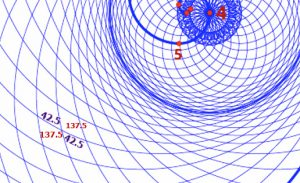them are the Purine groups that divide into 888 functional group nucleons and 629 sidechain nucleons and thus 1517 total nucleons. While (888 + 629) = 1517 = (37 x 24) + (37 x 17) = (37 x 41), the names Naftali, Benjamin, and Joseph = 888; and Shimon, Gad, and Joseph = 629. A similar breakdown occurs with the set of Pyrimidines that sum to (37 x 22) functional groups nucleons and to (37 x 22) sidechain nucleons for a total of (37 x 44) nucleons. Each group corresponds to the sum of the Names Dan, Asher, and Naftali. Since (41 + 44) = 85, altogether the Purines and Pyrimidines equal (37 x 85), as in the 85o complementary angle to the rotation of the Phi (φ) angle (137.5o) in the circle.

There is no question about the significance of the number/concept of 37 in the grand or cosmic schematic of our universe, we just need to understand it on a deeper level. All the names of the 12 sons were chosen for assorted spiritual reasons, yet they align cosmically so that Yehuda and Gad equal (30 + 7) = 37; and Levi, Gad and Dan equal (46 + 7 + 54) = 107, as in the Event Horizon that is also (54 x 107); Levi, Gad, Dan, and Yehuda equal (46 + 7 + 54 + 30) = 137, as in the Fine Structure Constant etc.; and Yehuda, Gad, and Dan equal (30 + 7 + 54) = 91, the unification in the Path of One; not to mention that Levi’s value of 46 matches the 46 Chromosomes and the cubit (46).

Does it all have something to do with the Primal Frequency (27.5 Hz) divided by 37 equaling (27.5/37) = .74, as in the 74 nucleons in all the functional groups of the amino acids? And do these both align with the 74 meridians in the human body?

Meanwhile, the sum of all 12 names of Jacob’s sons is 3182, or 3000 plus 182, the numerical value of Jacob (יעקב), and 3182/37 = 86, the numerical value of Elohim (אלהים).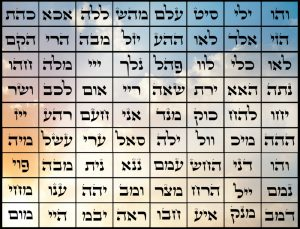The DNA connections to 37 are also most notably connected to the 37 values among the 72 Triplets that are less than 72, out of the 1200 possibilities. So, it is fitting that the 37th Triplet (אני), the middle Triplet of the 72 Triplets has a value of 61, the 18th Prime Number, the value of the Hebrew word for life, chai (חי), whose square root is 4.2426, as in Moshiach Ben David and the YHVH (יהוה). The value of the 37th Triplet (אני) is also the numerical difference (424363) = 61, between Moshiach Ben David and H’Moshiach, the two aspects of the Cosmic Consciousness embedded in the Spherical Time circumference (36304.24470).

This is significant because the 20 amino acids appear as products in the 64 Codons a total of 61 times.

Furthermore, 37 is the value of the back of the Name YHVH in the Aspect of Binah (יוד־הי־ואו־הי) in the same way that 19 is the back of the Name YHVH in the Aspect of Zeir Anpin or Man (יוד־הא־ואו־הא) of value 45, like Adam and Eve.Moreover, the ordinal or inner value of one of the 3 duplicate Triplets (לאו) is 19 which perfectly suits its standard value of 37, giving the two duplicate Triplets the complete value of 112, as in the 112 Essential Triplets and 112 chakras. Meanwhile, the first duplicate Triplet (והו) pair has a complete value of 68, as in chaim, Life. Since the 3rd duplicate set (מבה) has an ordinal value of 20, like the 20 amino acids, the total ordinal values for the 3 sets are also 112, and the complete value for all 3 sets is 314 or Pi (π).

Those 20 amino acids chains combine in myriad complex ways, yet nothing happens with them until they fold up into specific shapes. Now, while we would like to think that this is a natural process, they only fold under certain specific conditions like a temperature of 37o C and there are over 10300 ways for a single set of these to fold, let along all the different types that it takes to form an organism and then an ecosystem.  It would take evolution longer than the age of the universe for this to happen even just once, and that is only after it miraculously assembled the individual amino acids from the remnants of the stars.

Could life have sprung from this hyper-complex process of upside-down logic, where chaos leads to fine organization, that took significantly longer than the age of the universe, and that occurred based on a series of sequential chance encounters that are more numerous than the atoms in the universe? Or is there a simpler solution, one that does not involve our ego.

Another paradox is that this complex perfect folding happens in mere milliseconds and we do not have a clue how or why they fold the way they do. They are obviously preprogrammed to do so and to form into strips or Chromosomes that are each 6 ft or 72 inches or 27.5φ2 inches long when unwound, yet Man assumes there is no programmer, like apps appearing out of thin air onto our phones.

The connections to 37 does not end with the essence of life or with the Torah. It extends deep into the Prime Numbers and into Pi and it all connects through the letter Alef (אלף) of numerical value 111 or (3 x 37). We know that the 27 letters in the Alef-bet and the 27 positions in the Essential Magic Cube of Creation are naturally arranged so that they form triplicate digits that are multiples of 111 and hence of 37. We also know that those 27 letters of 9 tiers add up to (111 + 222999) = 4995, like the 4 nucleotides in our DNA whose molecular weight is 499.5. So, it is astounding that the sum of the set of the (111th, 222nd, 333rd… and 999th) Prime Numbers, gives us the palindromic result (607 + 1399… 7907) = 37073, as in the (37 x 73) structure and value of the first verse of the Torah. Moreover, it is phenomenal that the locations within Pi (3.14159265…) that we first encounter each of the numbers in this same set of 9 triple rep-digits (111, 222,… and 999) sum up to (153 + 1735…762) = 16012, and that when we add its reversed digits, they too arrive at (16012 + 21061) = 37073.

 Nth Prime # Prime Pi Location 111 607 153 222 1399 1735 333 2239 1698 444 3119 2707 555 4019 177 666 4973 2440 777 5903 1589 888 6907 4751 999 7907 762 4995 37073 16012 135 x 37 21061 37073

Adding the reverse of the digits is not arbitrary because the difference between these specific 9 prime numbers and these specific 9 Pi (π) locations is 21061, which just happens to be a numeric combination of the 210 height of the Tower of Truth and Sun Pyramid, and the value (61) of the 37th Triplet (אני) of the 72 Triplets deeply integrated into that Pyramid, and as in the Name Ani (אני) from “I am G-d” found in Ezekiel 37 and the prophecy of restoring life to the dry bones. It may also be a reference to the 61 letters in the 22 Names of the letters in the Alef-bet.

Moreover, 210 is the 20th Triangular Number, as in (3 + 7 + 0 + 7 + 3) = 20, like the 20 amino acids, and like (3 x 7 x 7 x 3) = 441, Emet, Truth. We see 210 again in the sum of (4995 + 16012) = 21007, and even 42 in (4995 + 37073) = 42068, this time coupled with 68, the value of chaim, life.

It gets better yet, or spookier. When we add our totals, thus all 27 numbers, they equal (4995 + 37073 + 16012) = 58080, which is exactly 2112 cycles of the Primal Frequency 27.5 Hz (58080/27.5 = 2112) with 73 being the 21st Prime Number and 37 being the 12th Prime Number.  Moreover, the palindromic 2112 has 28 factors like the 28 letters in the Torah’s first verse.  And just as (16 + 12) in 16012 equals 28, (21 + 61) in 21061 equals 82, which perfectly aligns with the 82 times the word-value 28 is found in the Torah.

And like the 3 rows of the amino acid matrix that sum to 1665, and the 3 rows of the normal magic 3 x 3 square that sum to 1665, and the 3 tiers of the Essential Magic Cube of Creation that each equal 1665, the 3 locations within the first 1000 digits of Pi (π) align with the triple rep-digits (111 + 555 + 999) likewise sum to 1665.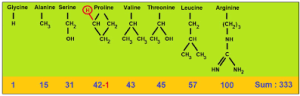The first 2 of those locations, corresponding to 666, (153 + 177) = 330, as in the base of the Sun Pyramid and (12 x 27.5), the Primal Frequency.

And all 9 triple rep-digit sequences appear within the Rumer Transformations, etc. in the combinations of our 20 amino acids. While it is obvious that they have the same Source Creator, is it possible the that the codes to life are found within the Torah and within the primal constant fields?

The value of all 3 of those 3 specific locations (153 + 177 + 762) = 1092 or twice 546, the sum of the initials of the 10 sefirot, brings up a prophecy and a challenge written almost 2000 years ago by Rabbi Shimon Bar Yochai in the Zohar:

### Knowledge for Our Time Only

In the Zohar, channeled and written in Aramaic nearly 2000 years ago by Rabbi Shimon Bar Yochai, in the section devoted to Torah portion Acharei Mot, Rabbi Shimon quotes Kohelet 1:7, “All the rivers run into the sea; yet the sea is not full….”  Then he immediately speaks about the end of days saying, “I wonder about people, that they have no eyes to see, no heart to observe, they do not know or pay attention to the wishes of their Master.  How asleep they are and do not awaken before the day will come when thick darkness will cover them; the owner of the deposit will demand his due accounting from them.”
Then after calling them fools and lovers of foolishness, the Zohar continues, “Come and see: Later generations will come when Torah will be forgotten among them, the wise will gather in their own place and there won’t be found anyone who can begin to explain and finalize [the Torah]. Woe to that generation. From here on, there will not be a generation like the present one until the generation of King Messiah (Moshiach), when knowledge will awaken in the world, as it is written, “For they shall all know Me, from the lowest of them to the greatest of them.” (Jeremiah 31:33).

Then later in the same section, in paragraph 303, we hear of the mysteries of the Holy Name and are told that they cannot be comprehended:
“…This is a matter that mankind cannot comprehend, nor can it rise in their minds, not to mention saying it with their mouths. Even supernal angels and the most sublime cannot comprehend it, as these matters are the mysteries of the Holy Name. There are 14,050,000 worlds dependent upon the stroke of the Alef (א) [which according to Rav Ashlag, refers to the stroke of the upper Yud (י) of the Alef], and the 72 Holy Names [Triplets ] are engraved in the impressed letters in them.  The high and low beings; heaven, earth, and the seat of glory of the King – are hanging from one side to the other [from the upper stroke to the lower stroke, Yud (י)] of the expansion of the Alef (א).  They sustain all the worlds and are the supports of the upper and the lower beings within the secret of wisdom.”

As Rav Yehuda Tzvi Brandwein of blessed memory explained to me several years ago the Upper Yud (י) corresponds to 14,050,000 worlds and filling in the blanks the lower Yud (י) corresponds to 300,109,265 worlds.

### It is the Mathematical constant Pi (π): 3.14159265…

What we see as numbers the tzaddikim of lore saw as camps of angels or worlds so for Rabbi Shimon, what we know as Pi (π) is a merging of two large aspects of the Creator. Applying Cosmic grammar to the language of Numbers this becomes easier to grasp.  Alef (אלף)  is the first letter of the Hebrew alphabet, but it is also the last in that the letter Alef (אלף) can have the numerical value of both 1 and of 1000, making it the lowest and the highest in their exponential gematria scale, representing the beginning and the end. As the Arizal explains the Alef (אלף) can thus also assume the value 1001.  Moreover, the Name of the letter Alef (אלף) when spelled out–a more potent version of the letter–takes on the numerical value of 111, so it is quite astonishing that when we divide 300109265 worlds by the total numerical value of all 28 letters of the Torah’s first verse (2701) we get 300109265/2701 = 111110 = 1001 x 111 = Alef (א) X Alef (אלף). Perhaps, this is why the cosmic Prime gematria cipher gives the word Echad (אחד) the value 28.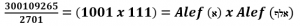Or viewed another way, the 300109265 worlds divided by Alef (א) X Alef (אלף) =2701, the Torah’s first verse, the 73rd Triangular Number. Another meaning to “He and His Name are One.” All aspects of the Creator.

## 300109265/(1001 x 111) = 2701.

This is why the Zohar section above begins with, “Come and see: The first subject of Torah we give to children is the Alphabet.”

The total standard gematria of the entire Torah is 21009826. We can call them values, fields, networks, or worlds; nevertheless, when we divide them by the 14,050,000 worlds, we get 1.495…, the standard gematria value of the sum of the 22 letters of Alef-bet.  Then if we divide the 300,109,265 worlds by 21009826, we get 14.28423372…, representing the 4 sets of 112 Triplets in so many ways and in so many cosmic networks (14, 28, 42, 33, 72).

### 64 Codons

The DNA programming is done using mRNA transcription and tRNA translation of the 4 nucleotides codes in a set of 43 or 64 Triplets or Codons that code for the 20 different Amino Acids. There are 64 possible permutations, or combinations of 3-letter nucleotide sequences that can be made from the 4 nucleotides. Within those 64 Triplets or Codons, the 4 nucleotides are repeated a total of (3 x 64) = 192 times.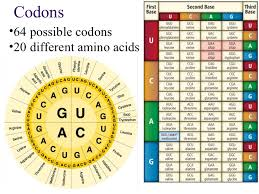When we add the Moshiach Ben David reference of ATCG (424) to the 192 times that these 4 nucleotides are repeated in our 64 codons it equals (424 + 192) = 616, the value of “HaTorah, The Torah.”

Applying small string gematria to the 4 nucleotides (ATCG) of DNA, they translate to 1423, which can be rearranged to 1234 or 1342 since the ATCG is in no particular order. The 4 nucleotides of RNA, AUCG would likewise be 1213, or 1312.

Using the same small gematria sequencing, their individual totals for the 4 DNA and RNA nucleotides are (1 + 2 + 3 + 4) = 10 and (1 + 2 + 1 + 3) = 7 respectively and together 17, as in Tov (good) and as in the value of the first Triplet (והו) of the 72 Names (Triplets), which is also 27.5/φ.

Adding up their individual digits values, the resultant sum of the 64 codons (Triplets) of DNA and RNA respectively would be (48 x 10) = 480 and (48 x 7) = 336. While 480 is the height of the Tower of Truth in feet, 336 matches the 336 letters in the 112 Triplets of the 4 Essential Elements (72 Triplets, 14 Triplets of the 42-Letter Name, 15 Triplets of the Shema, and the 11 Triplets of Bereshit).

Since the 64 codons code for 20 amino acids, it is not so surprising that (336 + 64) = 202, as in the base area of the Tower of Truth and the Holy of Holies.

### DNA and the 4 Hebrew Letters

The 4 DNA nucleotides (ATCG) are represented by the 4 Hebrew letters (אתכג).

The letter Alef (א) for Adenine is found 27059 times in the Torah, slyly referring to the 27.5 Hz Primal Frequency and the value 9 to which it is connected. It represents 8.8775% of the Torah, as in 5778 backwards.

The letter Tav (ת) for Thymine on the other end of the spectrum is found 17950 times, giving it 5.889% of the total letters in the Torah, or 5.778 plus .111, as the Name of the letter Alef (א).

When they are added together, the two bookend letters represent 14.7…% of the Torah, as in Jacob’s age (147). When the letter Caf (כ) for Cytosine, which is found 8610 times, is added they equal 17.5…% of the Torah, as in Abraham’s age (175).

Then finally when the 4th of the 4 letters that represent the 4 nucleotides in our DNA, the letter Gimmel (ג) for Guanine found 2109 times is added, altogether they are found 55728 times in the Torah and represent 18.2832% of it, as in the Jacob’s Name (182), and as in the 832 years that the First and Second Holy Temples stood. It is also the 832 years that stretches from the flood in 1656 to the death of Moses when the Israelites entered Israel in 2488 at the end of the 26 generations, 112 years before 2600.

The letter pair, Alef (א) and Gimmel (ג) are found respectively 27059 and 2109 times, hinting at 275 and 210 from the Tower of Truth, coupled with the value “9” that is key to frequency 27.5.

The number of Cafs (כ), Tavs (ת), and Gimmels (ג), representing the Sun, Moon, and Jupiter, nearly balance out the Alefs (א) in the Torah, 28869 vs 27059. The difference between them is 1610, as in highest Name Ehyeh (אלף־הי־יוד־הי), and as in the 4th and 7th levels of the 42-Letter Name that sum to (704 + 906) = 1610.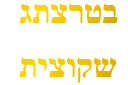That the 4 letters have a nominal value of 424 and that their total percent of the Torah is 18%, whose square root is 4.24 is cosmically telling enough, but that the square root of their exact 18.2832% is 4.275, as in the Primal Frequency 27.5 Hz and that (4 x .275) = 1.1, the other Primal Frequency, explains a lot more. This must all be balanced out with the 90,100 Yuds (י), Heis (ה), and Vavs (ו) in the Torah, as in 90100 being the 424th Triangular Number. Remember, it is a commandment to not change a single letter.  That extends to Man’s DNA as well, despite the intentions of the MRNA-1273 vaccine.

There is a reason the Torah needed to be preserved perfectly intact for us today, despite Man’s constant desire to rewrite all that came before him.

There is a Triplet amongst the 72 Triplets that stands outs from all the others because of its simplicity, purity, and all the empty space that surrounds it. It is the 22nd Triple (ייי) or 103, which is 1000 when knocked (multiplied) together.  Using the definition of the Arizal, it is also equal to 8000 when the expanded Name of the letter (יוד) is utilized.Since we can numerically translate the nucleotide letter C (כ) or 20 to the Name Yud (יוד) in our DNA, the corresponding translated codon (triplet) in both our DNA and RNA sequences is CCC or (ייי). With an astronomically high statistical improbability the codon CCC is also the 22nd Triplet in its matrix. And when the letter Caf (כ) is multiplied or knocked together, it too equals 203 or 8000. Of all the 64 codons in our DNA, it is the 22nd one that aligns with the 72 Triplets. Being the 22nd codon of 64, means that it is followed by 42 codons, and proceeded by 21. A most beautiful and Divine design indeed!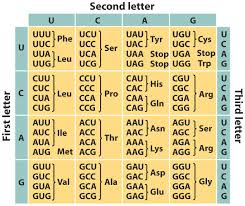We know that the 4 nucleotides in our DNA add up to 424, Moshiach Ben David, the Cosmic Consciousness, but with RNA and the addition of Uracil there are 5 nucleotides in total. When we add up all 5 nucleotides in our genetic codons (triplets), we get 425, the exact numerical value of the 8th Triplet (כהת) of the 72 Names.  And of course, this 8th Triplet, which entwines our genetics with this branch of the 5 Essential Elements of Creation, the 72 Triplet Matrix, is the last of the Matrix first row, meaning it is followed by the 8 x 8 foundation square of 64 Triplets, whose total value is 8000, like the product of the letters of the Sun (כ) and the Moon (ת), 8000, which are the first and last letters in the 8th Triplet (כהת) and are 2 of the 4 letters in our DNA (ATCG) that sum to 420.

Moreover, the 5 nucleotides in our DNA and RNA are each utilized 48 times to make the 64 codons. Altogether that is (425 x 48) = 20,400, once again as in Caf (כ) and Tav (ת).

The total for RNA alone is 1200, and the average for the codons of the DNA is exactly 318, as in the 318 men that Abraham took into battle against the 4 Kings in Genesis 14.

One Source.

### The Music of the Spheres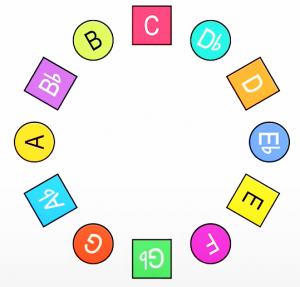The 12 Lunar Months with their referential YHVH (יהוה) in the 363 Day Cosmic Calendar correspond to the 12 musical notes that are based on the Primal Frequency (27.5 Hz) and that are arranged in a circle of 12.  It almost seems perfectly logical as the pictured circle of fifths is based on the number 5 like Phi (φ) and like the Prime Frequency 27.5 Hz.

There are 6 basic geometric patterns or shapes that connect them, like the distribution of the Planetary Letters associated with the 12 Moons of the 12 poles in the 6 Elliptical Orbits of the Cosmic Wheel and their associated elements of fire, water, earth, and air. They form triangles, squares, hexagons, dodecahedra, or stars that describe all the musical scales, intervals, chords, and progressions.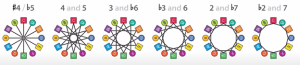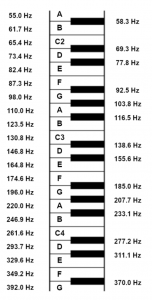A harmonic series (also called an overtone series) is the sequence of frequencies, musical tones, or pure tones in which each frequency is an integer multiple of a fundamental, in other words exact intervals based on the Fundamental Frequency 27.5 Hz, 27.5 vibrations/second, the melodic Voice of the Creator that reverberates through the Aether.

The note A begins at a frequency of 27.5 Hz and it follows an exponential scale of (27.5 x 2n) starting with A0, connoting octave 0, and advancing through 55, 110, 220, 440, and 880… as the higher octaves of note A. These are all phase points or rungs in the cosmic ladder between the Primal Frequencies 27.5 Hz and 1.1 Hz.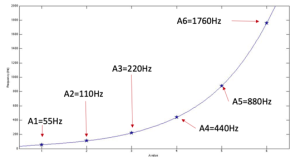The circle tones or frequencies that is music to our ears is arranged in a circle of fifths (1/5) whereby each note is 1/5th the prior going in a clockwise motion. In the diagram below the inner circle are the Major chords; the middle circle are the Minor chords; and the outer circle are the Diminished chords. The same 1/5th pattern holds for each circle, just like the 58 words, verses, and letters in the Torah that diminish by a factor of 1/5 until they reach 1. If they keep going 18 times, they reach 1024 x 10-10 elements, matching the 1024 vertices in the word value hypercube network of the Torah.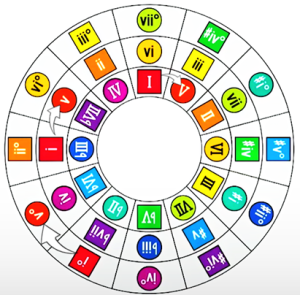The number 5, or the square root of 5, is at the basis of the Phi (φ) field, and thus we see spiraling in the opposite or counterclockwise direction the Phi (φ) angular progression of the notes from Major C – Minor C – Diminished C, and all the other notes as well. Three (3) tiers that progress by 1/5th is one direction along each tier, and simultaneously outward by Phi (φ) in the opposite direction. For more details on Music Theory check out Mike George’s videos.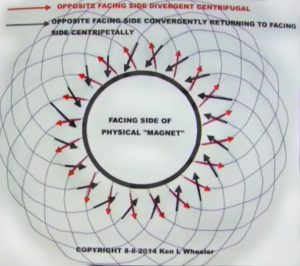The musical scale is divided into 12 notes that seem to work most pleasingly together in specific relationships and these are divided into an octave—8 relationships—that keep repeating with higher and higher octaves, each doubling the prior scale, or alternatively by halving.

Of the 27 letters of the Alef-bet there are only two letters that are curved, the 20th letter Resh(ר), and letter Caf (כ) of numerical value 20. Together their value is 220, and the typical musical scale begins at 220 Hz and thus the next octave (8) begins with 440 Hz. If we were to divide that 220 Hz between the octaves, we would get 8 evenly spaced increments of 27.5 Hz.  In other words, 220 Hz = (8 x 27.5) = 1 Octave, and our musical scall like the cosmos themselves is based on the Primal Frequency 27.5 Hz.

The most pleasing tonal relationship is that of the Perfect Fifth (P5) which is 1.5 times 220 Hz or 330 Hz, a musical/mathematical relationship of 3:2.  This Perfect Fifth (P5) or 330 Hz is, as we know, the base measure of the Pyramid of the Sun based on the Tower of Truth, or (12 x 27.5). It is also the perimeter of the 12 edges of a cube of 1 cubit. Perhaps the reason the Perfect Fifth (P5) is so pleasing is because there are 12 notes in a full cycle or scale and 330 Hz represents the first full cycle.

Another extremely pleasing note is the Major Third (M3) at 275 or 10 x 27.5 Hz. It is a C# (C-sharp) and also a Db (D-flat).

The Major Second (M2) is 247.5 Hz or (220 + 27.5 Hz), which is thus (9 x 27.5). It is interesting that this is so close to 248, Abraham, the 248 dimensions of the E8 Lattice, and the 248,000φ elements in the Torah, etc.  The Major Seventh (M7) is 412.5 Hz, or 3 intervals of the Phi (φ) angle (137.5o).

Not all 12 notes can work out to be exactly 27.5 Hz apart, given that the Octave (8) spacing is for (8 x 27.5). For example, the Tritone is 308 Hz which works out to 22 Hz less than 330 Hz and the minor Sixth (m6) is 352 or 22 Hz more than 330 Hz.  Together though, they are (308 + 352) = (2 x 330). Our first association is naturally to the 22 Hebrew letters but given that the other Primary Frequency associated with 27.5 Hz is 1.1 Hz and that they are both pulsed in 11 beats, this relationship probably has more to do with their phase alignment. To equalize the notes so that the relationships harmonize (more or less) between keys, all the values change to varying degrees, which is called just intonation in 12-part equal temperament.

A harmonic series or overtone series is the sequence of frequencies, musical tones, or pure tones in which each frequency is an integer multiple of a fundamental, in other worlds exact intervals based on the fundamental frequency 27.5 Hz, 27.5 vibrations/second, the Voice of the Creator.

#### 1 – 2 – 4 – 8 – 16

1 is 27.5, or 1 interval; 2 is 55, or 2 intervals; 3 is 82.5, or 3 intervals; 4 is 110 Hz, or 4 intervals; and 5 is 137.5, or 5 intervals; and 6 is 165, or 6 intervals; and 7 is 192.5, or 7 intervals; and 8 is 220 Hz, or 8 intervals; and 9 is 247.5 Hz, or 9 intervals; and 10 is 275 Hz, or 10 intervals; and 11 is 302.5, or 11 intervals; and 12 is 330 or 12 intervals; and 13 is 357.5 or 13 intervals; and 14 is 385 or 14 intervals; and 15 is 412.5 Hz or 15 intervals; and 16 is 440 Hz or 16 intervals…

#### 3 – 6 – 9

1 is 27.5, or 1 interval; 2 is 55, or 2 intervals; 3 is 82.5, or 3 intervals; 4 is 110 Hz, or 4 intervals; and 5 is 137.5, or 5 intervals; and 6 is 165, or 6 intervals; and 7 is 192.5, or 7 intervals; and 8 is 220 Hz, or 8 intervals; and 9 is 247.5 Hz, or 9 intervals; and 10 is 275 Hz, or 10 intervals; and 11 is 302.5, or 11 intervals; and 12 is 330 or 12 intervals; and 13 is 357.5 or 13 intervals; and 14 is 385 or 14 intervals; and 15 is 412.5 Hz or 15 intervals …

The 5th interval, or the number 5 integer field of 137.5 Hz is the Phi (φ) angle (137.5o) at the center of Spherical Time. There is some intuitive sense to this in that Phi (φ) or 1.6180339887… is derived directly from the number 5, as in the 5th interval.  This is the essence of the 5 Books of Moses and the 5 Essential Elements of Creation built into those 5 Books.

#### 5 is 137.5, or 5 intervals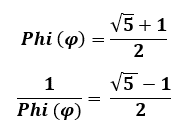#### Major Second (M2), Major Third (M3), Major Seventh (M7)

1 is 27.5, or 1 interval; 2 is 55, or 2 intervals; 3 is 82.5, or 3 intervals; 4 is 110 Hz, or 4 intervals; and 5 is 137.5, or 5 intervals; and 6 is 165, or 6 intervals; and 7 is 192.5, or 7 intervals; and 8 is 220 Hz, or 8 intervals; and 9 is 247.5 Hz, or 9 intervals, the Major Second; and 10 is 275 Hz, or 10 intervals, the Major Third; and 11 is 302.5, or 11 intervals; and 12 is 330 or 12 intervals; and 13 is 357.5 or 13 intervals; and 14 is 385 or 14 intervals; and 15 is 412.5 Hz or 15 intervals, the Major Seventh

#### Divine Intervals

The intervals of 9, 13, and 14 are associated with 248 Hz, as in Abraham; 358 Hz, as in Moshiach or Cosmic Consciousness; and 385 Hz, as in the Schechinah, the Divine Presence.

1 is 27.5, or 1 interval; 2 is 55, or 2 intervals; 3 is 82.5, or 3 intervals; 4 is 110 Hz, or 4 intervals; and 5 is 137.5, or 5 intervals; and 6 is 165, or 6 intervals; and 7 is 192.5, or 7 intervals; and 8 is 220 Hz, or 8 intervals; and 9 is 247.5 Hz, or 9 intervals; and 10 is 275 Hz, or 10 intervals; and 11 is 302.5, or 11 intervals; and 12 is 330 or 12 intervals; and 13 is 357.5 or 13 intervals; and 14 is 385 or 14 intervals; and 15 is 412.5 Hz or 15 intervals …

The Cosmos operates in perfect harmony.  We are the only ones that ignore it.

### The Voice of the Creator

The word emor (אמר) means “said” and it has a complete gematria of 275, as in the measure of the cubit (27.5”) and the Primal Frequency (27.5 Hz). Speaking in the proper frequency is certainly important, and perhaps this is why the word emor (אמר) is found 84 times in the Torah, as in the connected Upper and Lower Names of 42 that can bring Pad, redemption (84) as Rav Brandwein explained.

The triplet emor (אמר) is found within words in the Torah 1419 times as in Exodus 14:19 where we find the 72 Triplets hidden in the Torah at the exact moment of the Splitting of the Red Sea.

“If you want to find the secrets of the universe, think in terms of energy, frequency, and vibration.”